NEET  >  31 Year NEET Previous Year Questions: Equilibrium - 1

# 31 Year NEET Previous Year Questions: Equilibrium - 1

Test Description

## 17 Questions MCQ Test Chemistry Class 11 | 31 Year NEET Previous Year Questions: Equilibrium - 1

31 Year NEET Previous Year Questions: Equilibrium - 1 for NEET 2022 is part of Chemistry Class 11 preparation. The 31 Year NEET Previous Year Questions: Equilibrium - 1 questions and answers have been prepared according to the NEET exam syllabus.The 31 Year NEET Previous Year Questions: Equilibrium - 1 MCQs are made for NEET 2022 Exam. Find important definitions, questions, notes, meanings, examples, exercises, MCQs and online tests for 31 Year NEET Previous Year Questions: Equilibrium - 1 below.
Solutions of 31 Year NEET Previous Year Questions: Equilibrium - 1 questions in English are available as part of our Chemistry Class 11 for NEET & 31 Year NEET Previous Year Questions: Equilibrium - 1 solutions in Hindi for Chemistry Class 11 course. Download more important topics, notes, lectures and mock test series for NEET Exam by signing up for free. Attempt 31 Year NEET Previous Year Questions: Equilibrium - 1 | 17 questions in 35 minutes | Mock test for NEET preparation | Free important questions MCQ to study Chemistry Class 11 for NEET Exam | Download free PDF with solutions
 1 Crore+ students have signed up on EduRev. Have you?
31 Year NEET Previous Year Questions: Equilibrium - 1 - Question 1

### In a buffer solution containing equal concentration of B– and HB, the Kb for B– is 10–10. The pH of buffer solution is : 

Detailed Solution for 31 Year NEET Previous Year Questions: Equilibrium - 1 - Question 1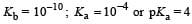For the buffer solution containing equal concentration of B and HB
pH = pKa + log 1
pH = pKa = 4
The octahedral complex ion [ CoCl2(NH3)4]+ i.e., tetra amminedichloro cobalt (III) ion exists as cis and trans isomers.

31 Year NEET Previous Year Questions: Equilibrium - 1 - Question 2

### Which one of the following molecular hydrides acts as a Lewis acid? 

Detailed Solution for 31 Year NEET Previous Year Questions: Equilibrium - 1 - Question 2

Boron in B2H6 is electron deficient

31 Year NEET Previous Year Questions: Equilibrium - 1 - Question 3

### The reaction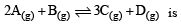begun with the concentrations of A and B both at an initial value of 1.00 M. When equilibrium is reached, the concentration of D is measured and found to be 0.25 M. The value for the equilibrium constant for this reaction is given by the expression 

Detailed Solution for 31 Year NEET Previous Year Questions: Equilibrium - 1 - Question 3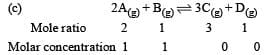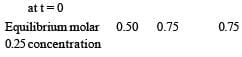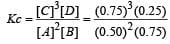31 Year NEET Previous Year Questions: Equilibrium - 1 - Question 4

A buffer solution is prepared in which the concentration of NH3 is 0.30M and the concentration of NH4+ is 0.20 M. If the equilibrium constant, Kb for NH3 equals 1.8 × 10–5, what is the pH of this solution ? (log 2.7 = 0.433). 

Detailed Solution for 31 Year NEET Previous Year Questions: Equilibrium - 1 - Question 4

Given [NH3] = 0.3 M, [NH4+] = 0.2 M, Kb = 1.8 × 10–5.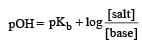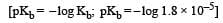∴  pKb = 4.74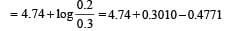= 4.56
pH = 14 – 4.56 = 9.436

31 Year NEET Previous Year Questions: Equilibrium - 1 - Question 5

Which of the following is least likely to behave as Lewis base ? 

Detailed Solution for 31 Year NEET Previous Year Questions: Equilibrium - 1 - Question 5

BF3 behaves as lewis acid.

31 Year NEET Previous Year Questions: Equilibrium - 1 - Question 6

In qualitative analysis, the metals of Group I can be separated from other ions by precipitating them as chloride salts. A solution initially contains Ag+ and Pb2+ at a concentration of 0.10 M. Aqueous HCl is added to this solution until the Cl concentration is 0.10 M. What will the concentrations of Ag+ and Pb2+ be at equilibrium?
(Ksp for AgCl = 1.8 × 10–10,
Ksp for PbCl2 = 1.7 × 10–5) [2011M]

Detailed Solution for 31 Year NEET Previous Year Questions: Equilibrium - 1 - Question 6

Ksp = [Ag+] [Cl] 1.8 × 10–10 = [Ag+] [0.1] [Ag+] = 1.8 × 10–9 M
Ksp = [Pb+2] [Cl]2 1.7 × 10–5 = [Pb+2] [0.1]2 [Pb+2] = 1.7 × 10–3 M

31 Year NEET Previous Year Questions: Equilibrium - 1 - Question 7

pH of a saturated solution of Ba(OH)2  is 12. The value of solubility product (KSP)of Ba(OH)2  is : 

Detailed Solution for 31 Year NEET Previous Year Questions: Equilibrium - 1 - Question 7

Given   pH = 12 or  [H+] = 10–12
Since, [H+] [OH] = 10–14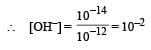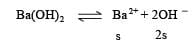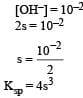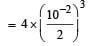= 5 × 10–7

31 Year NEET Previous Year Questions: Equilibrium - 1 - Question 8

Equimolar solutions of the following substances were prepared separately. Which one of these will record the highest pH value ? 

Detailed Solution for 31 Year NEET Previous Year Questions: Equilibrium - 1 - Question 8

(AlCl3, LiCl & BeCl2) ) all these solutions are acidic due to cationic hydrolysis, where as BaCl2, is salt of strong base and strong acid, hence its solution will almost neutral i.e., pH ≈ 7.

31 Year NEET Previous Year Questions: Equilibrium - 1 - Question 9

Buffer solutions have con stant acidity and alkalinity because 

Detailed Solution for 31 Year NEET Previous Year Questions: Equilibrium - 1 - Question 9

Lets take an example of an acidic buffer CH3COOH and CH3COONa.when few drops of HCl are added to this buffer, the H+ of HCl immediatly combine with CH3COO  ions to form undissociated acetic acid molecules. Thus there will be no appreciable change in its pH value. Like wise if few drops of NAOH are added, the OH – ions will combine with H+ ions to form unionised water molecule. Thus pH of solution will remain constant.

31 Year NEET Previous Year Questions: Equilibrium - 1 - Question 10

Given that the equilibrium constant for the reaction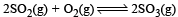has a value of 278 at a particular temperature. What is the value of the equilibrium constant for the following reaction at the same temperature ?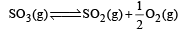[2012 M]

Detailed Solution for 31 Year NEET Previous Year Questions: Equilibrium - 1 - Question 10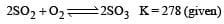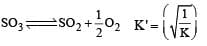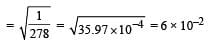31 Year NEET Previous Year Questions: Equilibrium - 1 - Question 11

Given the reaction between 2 gases represented by A2 and B2 to give the compound AB(g).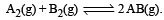At equilibrium, the concentration
of A2 = 3.0 × 10–3 M
of B2= 4.2 × 10–3 M
of AB = 2.8 × 10–3 M
lf the reaction takes place in a sealed vessel at 527°C, then the value of KC will be : [2012 M]

Detailed Solution for 31 Year NEET Previous Year Questions: Equilibrium - 1 - Question 11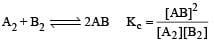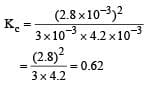31 Year NEET Previous Year Questions: Equilibrium - 1 - Question 12

Identify the correct order of solubility in aqueous medium:    [NEET 2013]

Detailed Solution for 31 Year NEET Previous Year Questions: Equilibrium - 1 - Question 12

Solubility of alkali metal is maximum among the following. Among ZnS (1.7 × 10–5) & CuS (8 × 10–37) ZnS has higher value  of Ksp.

31 Year NEET Previous Year Questions: Equilibrium - 1 - Question 13

Which of these is least likely to act as Lewis base?    [NEET 2013]

Detailed Solution for 31 Year NEET Previous Year Questions: Equilibrium - 1 - Question 13

BF3 is Lewis acid(e pair acceptor)

31 Year NEET Previous Year Questions: Equilibrium - 1 - Question 14

The values of Ksp of CaCO3 and CaC2O4 are 4.7 × 10–9 and 1.3 × 10–9 respectively at 25°C. If the mixture of these two is washed with water, what is the concentration of Ca2+ ions in water?     [NEET Kar. 2013]

Detailed Solution for 31 Year NEET Previous Year Questions: Equilibrium - 1 - Question 14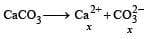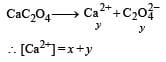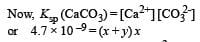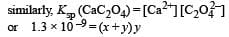On solving, we get [Ca2+] = 7.746 × 10–5 M

31 Year NEET Previous Year Questions: Equilibrium - 1 - Question 15

At 100°C the Kw of water is 55 times its value at 25°C. What will be the pH of neutral solution? (log 55 = 1.74) [NEET Kar. 2013]

Detailed Solution for 31 Year NEET Previous Year Questions: Equilibrium - 1 - Question 15

Kw at  25°C = 1 × 10–14 At 25ºC

Kw = [H+] [OH] = 10–14 At 100°C (given) Kw = [H+] [OH] = 55 × 10–14
∵ for a neutral solution [H+] = [OH]
∴ [H+]2 = 55 × 10–14
or [H+] = (55 × 10–14)1/2
∵  pH = – log [H+]
On taking log on both side – log [H+] = –log (55 × 10–14)1/2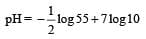pH =  – 0.87 + 7
=  6.13

31 Year NEET Previous Year Questions: Equilibrium - 1 - Question 16

Th e dissociation constan t of a weak acid is 1 × 10– 4. In order to prepare a buffer solution with a pH = 5 the [Salt]/[Acid] ratio should be [NEET Kar. 2013]

Detailed Solution for 31 Year NEET Previous Year Questions: Equilibrium - 1 - Question 16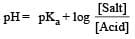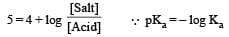Given  Ka =  1 × 10– 4
∴ pKa =  – log (1× 10– 4) =  4
Now from Handerson equation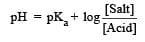Putting the values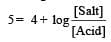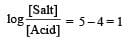Taking antilog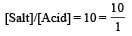31 Year NEET Previous Year Questions: Equilibrium - 1 - Question 17

Accumulation of lactic acid (HC3H5O3), a monobasic acid in tissues leads to pain and a feeling of fatigue. In a 0.10 M aqueous solution, lactic acid is 3.7% dissociated. The value of dissociation constant, Ka, for this acid will be: [NEET Kar. 2013]

Detailed Solution for 31 Year NEET Previous Year Questions: Equilibrium - 1 - Question 17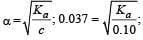Ka = (0.037)2 x 0.10 = 1.37 ×10–4

## Chemistry Class 11

204 videos|320 docs|229 tests
 Use Code STAYHOME200 and get INR 200 additional OFF Use Coupon Code
Information about 31 Year NEET Previous Year Questions: Equilibrium - 1 Page
In this test you can find the Exam questions for 31 Year NEET Previous Year Questions: Equilibrium - 1 solved & explained in the simplest way possible. Besides giving Questions and answers for 31 Year NEET Previous Year Questions: Equilibrium - 1, EduRev gives you an ample number of Online tests for practice

## Chemistry Class 11

204 videos|320 docs|229 tests

### How to Prepare for NEET

Read our guide to prepare for NEET which is created by Toppers & the best Teachers